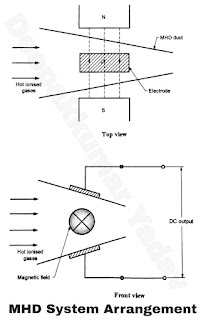# Working Principle of MHD Generator

Working Principle of MHD Generator

An MHD generator like a turbo generator is an energy conversion device. It can be used with any high temperature heat source like chemical, nuclear, solar energy etc. In an MHD system, hot gases act as the conductor. When gases are heated to sufficient temperature, one or more of the valance electrons are expelled from their orbit in which they are revolving. This ionises the gas i.e. the neutral atoms are split into positive and negative ions. These ions are the electrical conductors. The gases are moved in a duct called the MHD duct at a very high velocity. The movement of these ions in the MHD duct in which a strong magnetic field is applied leads to the electromagnetic induction of an e.m.f in a pair of electrodes which are placed in a direction perpendicular to both the directions of the flow of the ionised gases and that of the magnetic field. The electrodes are connected to the external circuit which drives a current in the external load.

One of the main practical problem encountered in this system is that, the ionisation temperatures of gases in their pure states are very high. Hence handling of these high temperatures will cause problem due to the limitations of materials. To get over this problem, the gas is seeded with small quantities of alkaline metals like sodium or potassium. Upon mixing, these ionisation temperature drops considerably in the range of 2300°C to 2700°C. Thus the problem of high temperatures requirement can be eliminated.

MHD System Arrangement :

Figure A & B shows the arrangement of and MHD generator. The three major components the MHD duct, the magnetic field and the electrodes. The Figure A & B shows the positions of the directions of the magnetic field, the direction of flow of the gases and the electrodes which are mutually perpendicular to each other.

The important quantities involved in MHD are :

Power generated = P

Electrical conductivity = U

Velocity of the gas = V

Magnetic field strength = B

Density of the gas = p

Length of duct = L /

The power generated by MHD generator is directly proportional to length of duct, electrical conductivity, the velocity of gas and square of the magnetic field strength while it is inversely proportional to the density of gas. Mathematically,

Power generated = P = ² L . Ω . V . Bρ   ...(1)Figure A
The power produced by the MHD system will be a DC. For power transmission, this DC power needs to be converted into AC which can be done by the use of an inverter.

Fig shows the principle components of a MHD system.

Thermal Efficiency : η = Work output / Heat supplied = (hh02) - (h04 h03) / (h01 h04)

Where the indicated enthalpies in Equation (2) are stagnation enthalpy. If V is the flow velocity, Stagnation enthalpy,  h= Static enthalpy, h + K . E. (V/ 2)

If a MHD generator works between the temperature limits of T= 3000 K and T= 3000 K, its Carnot efficiency, will be,

η= 1 - T/ T= 1 - 300 / 3000 = 0.9 or 90%

Practically, the efficiency obtained is much less for the following reasons :

If the temperature of the gas falls below 2000°C, the electrical conductivity of the gas reduces drastically. It is due to the fact that the electrons combine with ions to form neutral atoms at lower temperatures. Thus the power output of MHD also reduces rapidly.

To avoid this problem, MHD system is used in conjunction with steam power plants. Initially, when the temperatures of the gases are high , the energy of the gases is utilized in the MHD system for direct conversion into electricity. When the temperature of the gases falls to a low value, the hot gases are used for steam generation. This steam is utilized to generate electric power in a conventional steam power plant. Thus the MHD generator is used as sopping unit and the steam power plant is used as a bottoming unit.